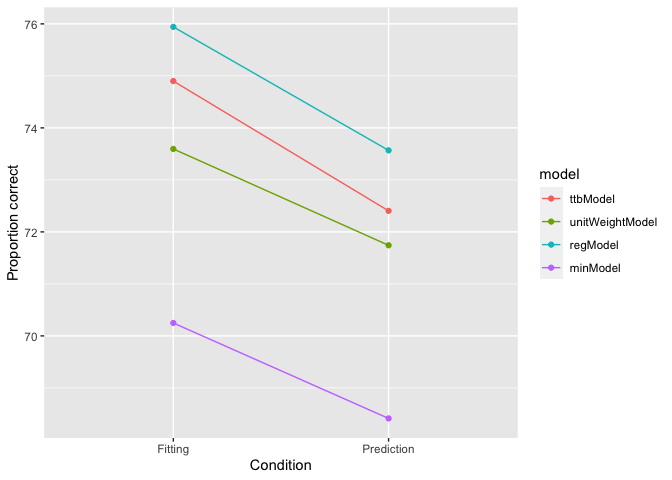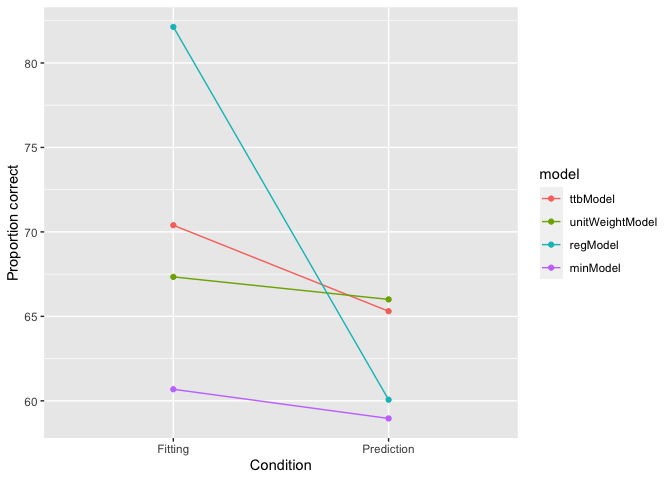# Comparing the performance of simple heuristics using the Heuristica R package

#### 2021-09-06

library(heuristica)

This document provides a simple example of how to compare the out-of-sample performance of different models in the heuristica package.

Replication

# Use this seed to exactly replicate my tables and graphs below.
#set.seed(3)
# Remove it to see a new sampling-- and whether the overall conclusions still
# hold.

Helper functions

First let’s load the heuristica package to get the heuristics we will compare. It also includes functions to calculate accuracy.

Let’s enter the models we want to test:

vec_of_models <-c(ttbModel, unitWeightModel, regModel, minModel)

Here’s a function that does cross-validation taking the vector of models, criterion column, columns to fit, the dataset, and the number of repetitions as input:

crossV <- function(vec_of_models, criterion_col, cols_to_fit, data, reps,training_proportion){
fitting <- vector()
prediction <- vector()
for(i in 1:reps){

#randomly sample training and test row indexes
train <- sample(1:nrow(data), nrow(data)*training_proportion)
test <- setdiff(1:nrow(data), train)

#create training and test set
training_set <- data[train,]
test_set <- data[test,]

# If a regression is overdetermined (e.g. has too many columns(), it will
# drop the right-most columns.  To instead make it drop random columns,
# we shuffle the column order.
shuffled_cols_to_fit <- sample(cols_to_fit)

models<-list()
y <- 0
for (mod in vec_of_models) { #fit the models to the training_set
y <- y+1
models[[y]] <- mod(training_set, criterion_col, shuffled_cols_to_fit)
}

#calculate percentage of correct predictions
fittingAccuracy <- percentCorrectList(training_set, models)
predictionAccuracy <- percentCorrectList(test_set, models)
fitting <- rbind(fitting,fittingAccuracy)
prediction <- rbind(prediction,predictionAccuracy)
}

results <- (rbind(colMeans(fitting),colMeans(prediction)))
rownames(results) <- c("Fitting","Prediction")
results
}

City population

Then we can just run this function to calculate predictive accuracy for different training and test set sizes. First let’s have the models predict the populations of 83 German cities using 9 binary cues. The criterion column may change depending on your data set, so set it correctly!

data("city_population")
data_set <- city_population
criterion_col <- 3
cols_to_fit <- 4:ncol(data_set)

Below we have the models train on 0.5 of the data (50%) and predict the other half, and we repeat this for 100 samples of splitting the data in half.

reps <- 100
training_proportion <- 0.5
results <- crossV(vec_of_models, criterion_col, cols_to_fit, data_set, reps,training_proportion)
round(results, 1)
##            ttbModel unitWeightModel regModel minModel
## Fitting        74.9            73.6     75.9     70.2
## Prediction     72.4            71.7     73.6     68.4

Finally, let’s plot the results:

library(ggplot2)
library(reshape)
rownames(results) <- c("Fitting","Prediction")
p <- melt(results)
## Warning in type.convert.default(X[[i]], ...): 'as.is' should be specified by the
## caller; using TRUE

## Warning in type.convert.default(X[[i]], ...): 'as.is' should be specified by the
## caller; using TRUE
colnames(p) <- c("condition","model","value")
ggplot(p, aes(x=condition, y=value, colour=model,group=model)) +
geom_line() +
geom_point() +
xlab("Condition") + ylab("Proportion correct")High school drop-outs

Now do the same analysis for the high school drop-out data set. It has 23 real-valued cues (rather than binary cues) for 63 Chicago public high schools.

Note that this data set has na’s, so we use na.omit to clean them because not all heuristics can handle them properly.

data(highschool_dropout)
data_set <- na.omit(highschool_dropout)
criterion_col <- 4
cols_to_fit <- 6:ncol(data_set)

reps <- 100
training_proportion <- 0.5
results <- crossV(vec_of_models, criterion_col, cols_to_fit, data_set, reps,training_proportion)

rownames(results) <- c("Fitting","Prediction")
p <- melt(results)
## Warning in type.convert.default(X[[i]], ...): 'as.is' should be specified by the
## caller; using TRUE

## Warning in type.convert.default(X[[i]], ...): 'as.is' should be specified by the
## caller; using TRUE
colnames(p) <- c("condition","model","value")
ggplot(p, aes(x=condition, y=value, colour=model,group=model)) +
geom_line() +
geom_point() +
xlab("Condition") + ylab("Proportion correct")Discussion

The performance of all models drops when they are predicting unseen data. In the city population dataset the rank-order of the models remains the same for fitting and prediction. However, when predicting high-school dropout some of the simple models (TTB and UnitWeightModel) outperform linear regression in prediction. These results suggests that different environmental structures (such as the number of cues in the environment) favor different strategies.

How would other models compare to take-the-best? Try some of the existing models in the heuristica package (e.g., logRegModel for logistic regression) or create your own model (see vignette on ‘how to make a heuristic’).